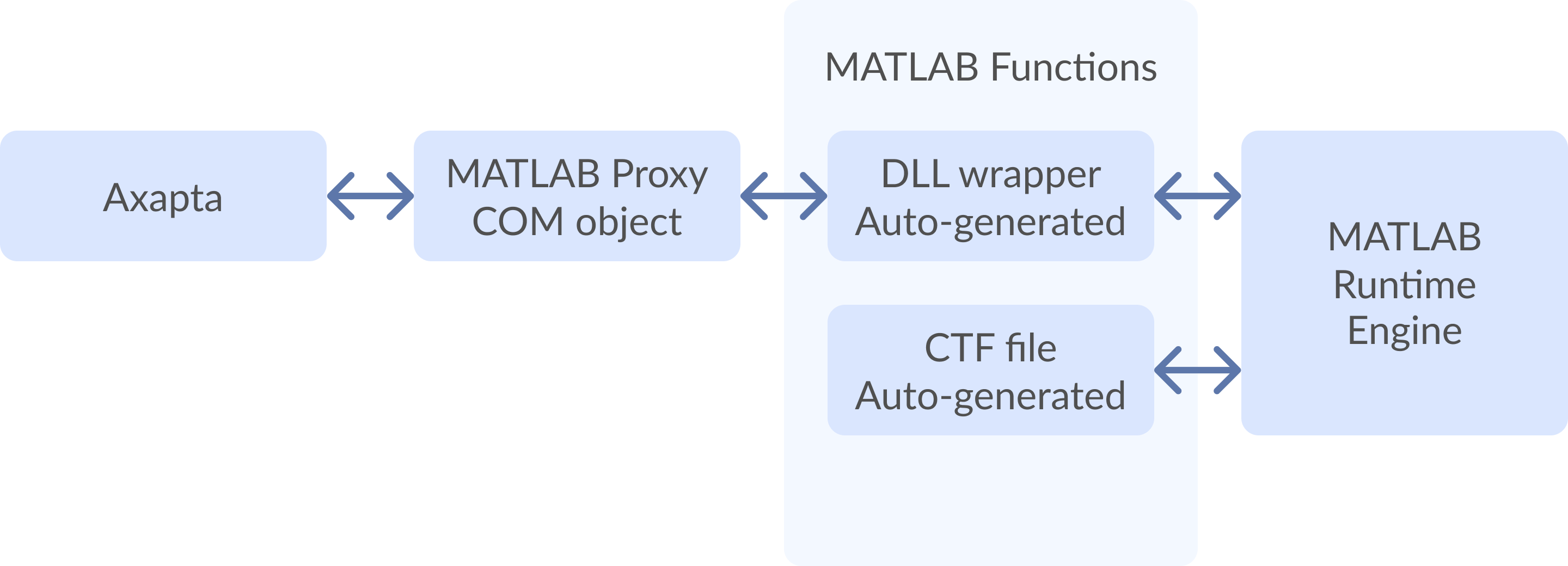# Matlab Integration with Microsoft Dynamics AX (Axapta)

## 1. Application Area

Matlab Integration solution is presented for Dynamics AX (Axapta) as a way to improve Dynamics AX (Axapta) computation performance significantly. It can be used for any performance critical calculation tasks.

An interface between Dynamics AX (Axapta) code and Matlab mathematical functions is implemented. Standard Dynamics AX (Axapta) doesn’t have built-in modules (classes) for this purpose, so own wrappers for Matlab-generated DLLs are necessary.

Matlab Integration solution provides a convenient and easy feature to call Matlab functions from Dynamics AX (Axapta) code. All required conversions for parameters and types are performed automatically.

## 2. What is Matlab?

Matlab® is a high-performance language for technical computing. It integrates computation, visualization, and programming in an easy-to-use environment where problems and solutions are expressed in familiar mathematical notation. Using Matlab, it is possible to solve technical computing problems faster than with traditional programming languages, such as C, C++, and FORTRAN.

Matlab provides a number of features for documenting and sharing a work. User can integrate his Matlab code with other languages and applications, and distribute own Matlab algorithms and applications.

## 3. Integration Idea

General idea is to implement a universal module that provides interface to Matlab features from Dynamics AX (Axapta). This module is a COM-object that can be used from any code such as C++, Visual Basic etc.

Matlab provides its own COM-objects that can be produced via Matlab COM-Builder tool. However, these objects are unusable for Dynamics AX (Axapta) because of some technical restrictions.

Dataflow diagram is shown in Figure 1.Figure 1: Dataflow diagram

Dynamics AX (Axapta) code uses Matlab Proxy Object that operates with Matlab function wrapper DLL. Matlab compiler produces that wrapper DLL automatically for user function. CTF file contains compiled function code that is executed by Matlab runtime engine.

Matlab Proxy module maintains universal object model that allows using of any Matlab function independently on its parameters, return values and types.

## 4. Performance

Performance test results shown in Table 1 illustrate a significant advantage of Matlab vs Dynamics AX (Axapta) code for arithmetic operations.

 Test Platform Integer Double Total Axapta 2.5 1260 6800 8060 Axapta 3.0 with debug mode 6890 10920 17810 Axapta 3.0 w/o debug mode 6180 10060 16240 Axapta 3.0 with “C” DLL 30 10 40 Axapta 3.0 with “C#” COM 30 20 50 Axapta 3.0 with COM connector 2070 6710 8780 XAL 2.8 1000 6000 7000 VBA macro (MS-Word 2003) 440 130 570 VC++ 6.0 (exe) 30 10 40 C# NET (exe) 40 10 40 MATLAB 7.01 N/A 20 20

Table 1: Performance test results

#### Remarks to the test results:

• “Integer” column means calculation with operand types of “Integer”, “Double” – “Real” (Axapta) or “Double” (C/C#).
• Figures in columns are in milliseconds, qty of iterations is 100000 * 10 = 1000000.
• Tests performed on PC AMD Athlon 2100+ RAM 1*256 DDR.
• Exe files, generated using VC++ 6.0 and C# NET, and corresponded DLLs, called inside Dynamics AX (Axapta), gave approx the same results. To improve performance with arithmetic operations in Dynamics AX (Axapta) we can transfer arithmetic routine in DLL written in C/C#. Overhead for DLL calls is 10-300 ms approximately.
• Dynamics AX (Axapta) 3.0 on arithmetic tests is approx 400 times slower than VC 6.0 and C# NET (EXE or DLL).
• Dynamics AX (Axapta) 3.0 on arithmetic tests is approx 30 times slower than VBA code.
• Dynamics AX (Axapta) 3.0 on arithmetic tests is approx 2.3 times slower than XAL 2.8 code.
• Dynamics AX (Axapta) 3.0 on arithmetic tests is approx 2 times slower than Dynamics AX (Axapta) 2.5.
• Debug mode in Dynamics AX (Axapta) 3.0 effects on performance results, but not much (10% approximately).
• Dynamics AX (Axapta) 3.0 on arithmetic tests is approx 1.85 times slower than Dynamics AX (Axapta) 3.0 code with COM connector.

## 5. Conclusion

Usage of Matlab Integration gains much better performance for mathematic operations in Dynamics AX (Axapta). It efficiently utilized the power Matlab suite and extends standard Dynamics AX (Axapta) functionality. Described approach has broad applicable area for implementation of our Business Optimization Solutions.